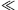Mathematical and Physical Journal
for High Schools
Issued by the MATFUND Foundation
 Already signed up? New to KöMaL?

#Problem P. 4211. (December 2009)

P. 4211. A current of I is flowing in a wire which is in the centre of an infinitely long straight metal tube. The radius of the tube is r the width of its wall is d (dr), and the young modulus of its material is E. By what amount does the radius of the tube change if a current of magnitude I is started in the tube which current is opposite to the current in the wire. Does the radius increase or decrease?

(5 pont)

Deadline expired on January 11, 2010.

Sorry, the solution is available only in Hungarian. Google translation

Megoldás. A sugár megnő $\displaystyle \Delta r=\frac{\mu_{0}I^2}{8\pi^2d\,E}$ értékkel.

### Statistics:

 22 students sent a solution. 3 points: 20 students. 1 point: 2 students.

Problems in Physics of KöMaL, December 2009